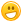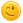# How do you ask a question in Python 3?

+5 votes
asked Jun 22, 2020
I'm coding a game, and I don't know how to get the program to let my player type in an answer. Can someone help me, please?

## 7 Answers

–1 vote
answered Jun 24, 2020 by (22,620 points)

You can search the Internet for various documentations and tutorials on Python.

One of them is on W3School that describes how to handle user input in Python:

https://www.w3schools.com/python/python_user_input.asp

commented Mar 11 by (1,090 points)
Why the dislikes?
0 votes
answered Jun 27, 2020 by (180 points)

For Example if you want the user to enter his/age you can prompt the user by using this line of code :

age = int(input("Enter your age : "))

for accepting the name and other details :

name = input('Enter your name : ')

–2 votes
answered Jul 2, 2020 by (100 points)

To ask a simple question like what school you go to, do this:

school = input("What school do you go to? : ")

print("You go to " , school)

If you want to ask a different question, just replace the variable with what the question is about,  replace the school question with yours then,  print you start of the answer.

Thx!

0 votes
answered Oct 6, 2020 by (440 points)
simple,if you need ask to the player "whats the name of your caracther" for example you can write:

print('Name of your character:')

name = input('')

print("the name of your caracter is {}").name #this line can be wrong, mayble the correct is this:print("the name of your caracter is {name}") i dont rememeber but this you dont use now
+1 vote
answered Oct 7, 2020 by (6,240 points)

In Python 2.7 =  username = raw_input("Enter username:") - This method is for Python2 which is approaching EOL.

In Python3 = username = raw_input("Enter username:")

Interestingly, Python will read the response as a string, so if you want to read a number and treat it as a number, I would recommend age = int(input("Enter your age : "))

0 votes
answered Oct 11, 2020 by (140 points)
player=input('enter anwer')

x=str (player)
0 votes
answered Mar 2 by (440 points)
Use the "input()" Function

Put the question in the parenthesis

(I recommend using a recent input variable such as "RecentImput" and putting this line of code:

RecentImput = input("Your question here")

Then use an if command to see if the input is any one of a few inputs you will allow)